### Home > A2C > Chapter 13 > Lesson 13.1.1 > Problem13-9

13-9.
1. Given the function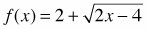, find each of the following. Homework Help ✎

1. The domain and range.

2. The inverse equation.

3. The domain and range of the inverse.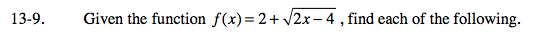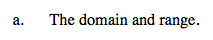Sketch a graph. What is the smallest x-value you can use before the number under the radical sign becomes negative?
What is the smallest y-value that can result from this equation?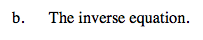$f^{-1}(x) = \frac{(x-2)^2}{2}+2$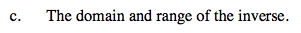Remember that in an inverse, x and y are interchanged. Reverse the domain and range you found in part (a).